Science, Maths & Technology

### Become an OU student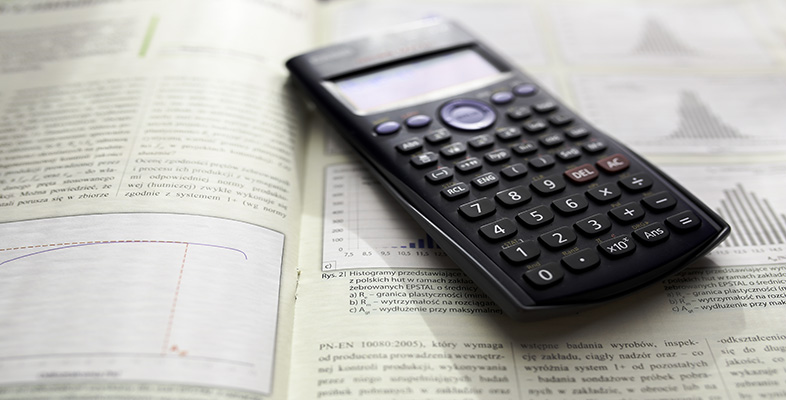Using a scientific calculator

Start this free course now. Just create an account and sign in. Enrol and complete the course for a free statement of participation or digital badge if available.

# 6.1 Using roots on your calculator

Just as there are keys on your calculator for entering powers, roots can also be entered directly. Square roots can be calculated using the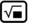key. For example,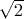can be entered using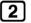. Cube roots are entered using the second function of this key. For higher roots, such as fourth or fifth roots you need to use the more general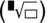template, which is the second function of the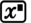key. This template is filled in by using the number and arrow keys (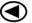and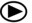) in a way similar to that used when the fraction template is completed.

## Activity 15 Calculating roots

1.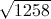2.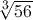3.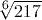4.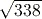1.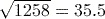(to 3 significant figures) using the key sequence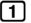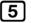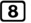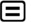.
2.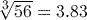(to 3 significant figures) using the key sequence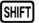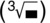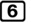.
3.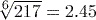(to 3 significant figures) using the key sequence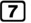.
4.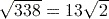or 18.4 (to 3 significant figures) using the key sequence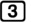(and usingto find the decimal result).
To find the decimal equivalent of an answer like this, you can use the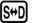orkeys that you used earlier to find the decimal forms of fractional answers.